Most Affordable JEE | NEET | 8,9,10 Preparation by Kota's Top IITian Doctor Faculties

# Wave Optics Class 12 Important Questions-Fully Solved Physics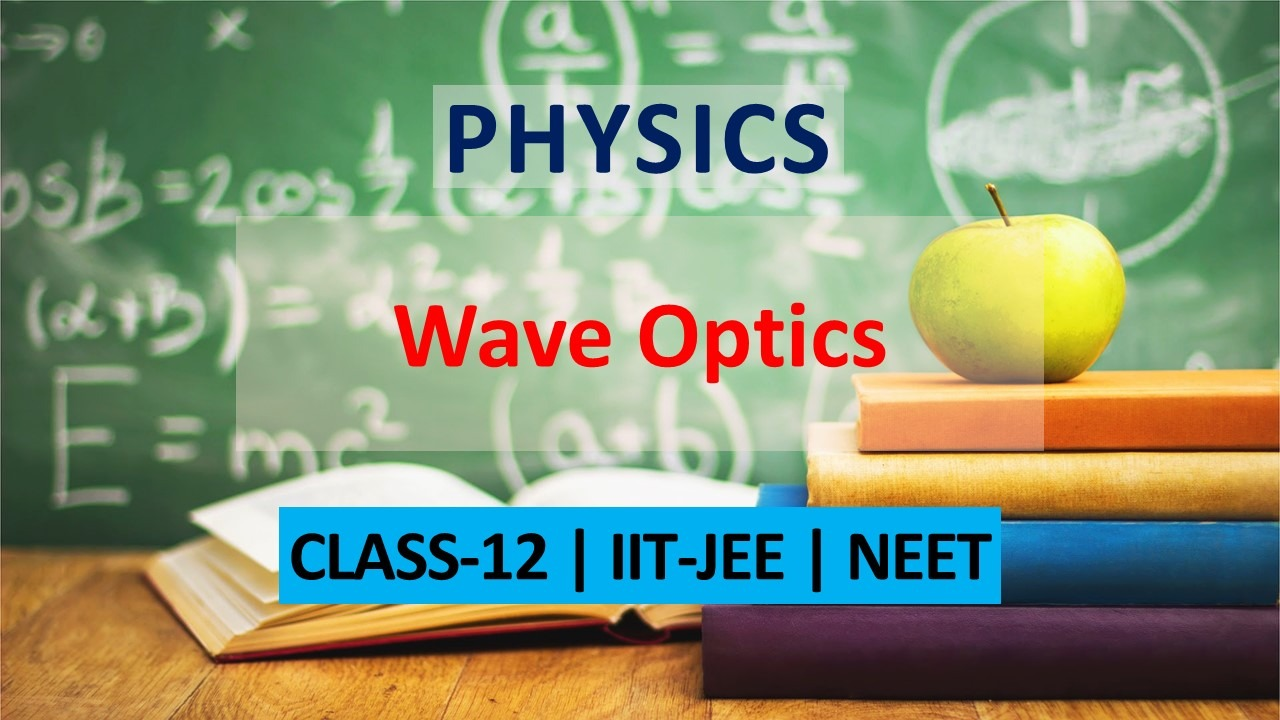Get important questions of Wave Optics Class 12 for Boards exams. Download or View the Question Bank for Class 12 having important questions to clear the concepts of wave optics. This question bank is designed keeping NCERT in mind and the questions are updated with respect to upcoming Board exams. You will get here all the important questions for class 12 Physics. Learn all the concepts of Wave optics with these questions and answers,along with it, you can find class 11 and class 12 comlete syllabus important questions question bank and notes. Click Here for Detailed Chapter-wise Notes of PHYSICS for Class 12th, JEE & NEET.
Q. State two conditions for sustained interference of light.
Ans. Conditions for sustained interference are as follows (i) The two sources of light must be coherent i.e. they should emit light waves of same wavelength or frequency with constant or zero phase difference. (ii) The two sources should preferably be monochromatic
Q. How is a wave front different from a ray?
Ans. The locus of all the particles of medium vibrating in the same phase is called wavefront. An arrow down normal to the wave front and pointing in the direction of propagation of disturbance represents a ray of light.
Q. What will be the effect on the interference fringes in a Young’s double slit experiment, if (i) Monochromatic source is replaced by a source of white light (ii) The screen is moved away from the slit ? Justify your answer
Ans. (i) When a source of white light is used in place of monochromatic light then central fringe is white. As blue colour has lowest wavelength so the fringes closest on both sides of central white fringe are blue and the farthest fringes are red. After a few coloured fringes, the pattern disappears. (ii) When screen is moved away from the slit, distance between the slits and screen (D) increases. As $\beta=\frac{\lambda D}{d}$ therefore width of fringes increases.
Q. What is a wave front ? What is the geometrical shape of a wave front of light emerging out of a convex lens, when point source is placed at its focus?
Ans. Wave front : It is defined as the locus of all the particles of a medium vibrating in the same phase at a given instant.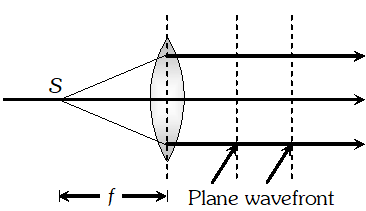Q. Two independent sources of light cannot be coherent, Why ? Or Two identical and independent sodium lamps acts as coherent sources ?
Ans. This is because, the light is emitted from individual atoms, when they return to ground state after being excited by heat or electric discharge. Even the smallest source of light contains billions and billions of such atoms which obviously cannot emit light waves in same phase. Such independent sources are called non coherent sources.
Q. Two identical coherent waves, each of intensity I, are producing an interference pattern. Write the value of the resultant intensity at a point of (i) Constructive interference and (ii) Destructive interference.
Ans. By using $I_{R}=I_{1}+I_{2}+2 \sqrt{I_{1} I_{2}} \cos \phi$
Q. Light of wavelength 600 nm is incident on a single slit of width 0.5 mm at normal incidence. Calculate the separation between two dark bands on either side of the central maximum, if the diffraction pattern is observed on a screen placed at 2 m from the slit.
Ans. Separation between two dark bands on either side of the central maxima = width of central maxima $=\frac{2 \lambda D}{d}$ Where $\lambda=600 n m=600 \times 10^{-9} \mathrm{m}$ $d=0.5 \mathrm{mm}=0.5 \times 10^{-3} \mathrm{m}$ and $D=2 \mathrm{m}$ Hence separation $\frac{2 \times 600 \times 10^{-9} \times 2}{0.5 \times 10^{-3}}=9.8 \times 10^{-3} \mathrm{m}=4.8 \mathrm{mm}$
Q. Two wavelengths of sodium light 590 nm, 596 nm are used, in turn, to study the diffraction taking place at a single at a single slit of aperture $2 \times 10^{-6} \mathrm{m}$. The distance between the slit and the screen is 1.5 m. Calculate the separation between the positions of first maximum of the diffraction pattern obtained in the two cases.
Ans. Position of $n^{\text {th }}$ secondary maxima on screen is given by $x=\frac{(2 n+1) \lambda D}{2 d}$ For first secondary maxima $n=1 \Rightarrow x=\frac{3 \lambda D}{2 d}$ With wavelength $\lambda_{1}$ position of first maxima $x_{1}=\frac{3 \lambda_{1} D}{2 d}$ $\Rightarrow x_{2}-x_{1}=\frac{3 D}{2 d}\left(\lambda_{2}-\lambda_{1}\right)$ $=\frac{3 \times 1.5}{2 \times 2 \times 10^{-6}}\left(596 \times 10^{-9}-590 \times 10^{-9}\right)$ $=6.75 \times 10^{-3} \mathrm{m}=6.75 \mathrm{mm}$
Q. Show graphically the intensity distribution of a single slit diffraction pattern. State with reason, how would the linear width of central maximum change if (i) Monochromatic yellow light is replaced with red light, and (ii) Distance between the slit and the screen is increased.
Ans. The intensity distribution curve for diffraction at a single slit is shown below.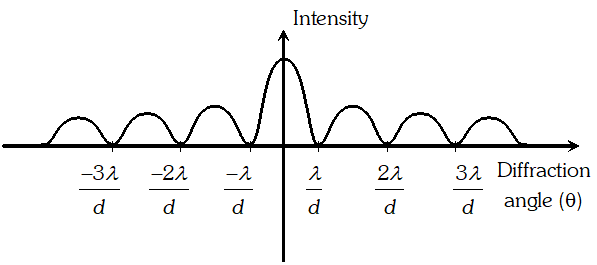Width of central maxima is $W=\frac{2 \lambda D}{d}$ (i) As $\lambda_{\text {red }}>\lambda_{\text {yollow. So width of central maxima }}$ increases when yellow light is replaced with red light (ii) When distance between the slit and the screen (D) is increased, the width of central maxima increases.
Q. What are coherent sources of light? Why is no interference pattern observed when two coherent sources are (i) Infinitely close to each other? (ii) For apart from each other ?
Ans. The sources of light, which emit continuously light waves of the same wavelength, same frequency and in same phase or having a constant phase difference are called coherent sources. As, fringe width $(\beta) \propto \frac{1}{\text { seperation between two sources }(\mathrm{d})}$ (i) When the two sources are placed infinitely close to each other, the fringe width becomes very large. Even a single fringe may occupy the screen. The interference pattern is not observable. (ii) As the distance between the sources is increased, the fringe width goes decreasing. At very large separation, it becomes too small too be detected.
Q. State Huygen’s postulates of wave theory. Sketch the wavefront emerging from a (i) Point source of light and (ii) linear source of light like a slit
Ans. Huygen’s postulates : (i) Every point on the given wave front acts as a fresh source of new disturbance, called secondary wavelets, travel in all directions with the velocity of light in the medium. (ii) A surface touching these secondary wavelets tangentially in the forward direction at any instant gives the new wave front at that instant. This is called secondary wave front. Wave fronts :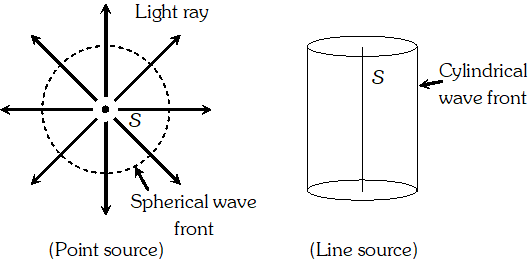Q. In young’s double slit experiment, deduce the condition for (i) Constructive, and (ii) Destructive interference at a point on the screen. Derive the expression for fringe width.
Ans. Consider two narrow slits $S_{1}$ and $\mathrm{S}_{2}$ acts like two coherent sources. Source S is placed on the perpendicular bisector of $\mathrm{S}_{1} \mathrm{S}_{2}$ and is illuminated with monochromatic light of wavelength Separation between the slits is d and separation between the plane of slits and screen is D.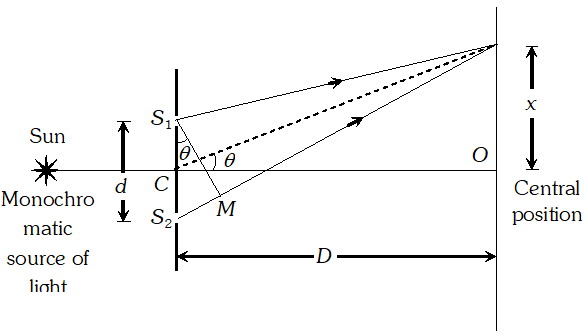Consider a point P on the screen at a distance x from central bright fringe O Draw a perpendicular $S_{1} M$ on $S_{2} P,$ so the path difference between the waves reaching at $P$ is $\Delta=S_{2} M=S_{1} S_{2} \sin \theta=d \sin \theta$ …(i) From $\Delta P C O \quad \sin \theta=\frac{O P}{P C} \approx \frac{O P}{C O}=\frac{x}{D}$ …(ii) From equations (i) and (ii) we get Path difference $\Delta=\frac{x d}{D}$ …(iii) (i) For constructive interference at $P$, $\Delta=n \lambda: n=0,1,2,3 \ldots$ Hence $n \lambda=\frac{x d}{D} \Rightarrow x=\frac{n \lambda D}{d},$ this is the position of $\mathrm{n}^{\text {th }}$ bright fringe on screen, from central bright fringe. (ii) For destructive interference at $P$ $\Delta=\frac{(2 n-1) \lambda}{2} ; n=1,2,3, \ldots$ Hence $\frac{(2 n-1) \lambda}{2}=\frac{x d}{D} \Rightarrow x=\frac{(2 n-1) \lambda D}{d}$ This is the position of $\mathrm{n}^{\mathrm{th}}$ dark fringe from central bright fringe. Fringe Width : In fringe pattern, so obtained, all the fringes are of equal width. Fringe width is the separation between two consecutive similar fringes on the screen. Hence fringe width $(\beta)=x_{n}-x_{(n-1)}$ Where $x_{n}=$ position of $\mathrm{n}^{\text {th }}$ bright or dark fringe from central position and $x_{n-1}=$ position of $(n-1)^{n}$ bright or dark fringe from central position If we consider bright fringes then $x_{n}=\frac{n \lambda D}{d}$ and $x_{n-1}=\frac{(n-1) \lambda D}{d}$ $\Rightarrow \quad \beta=\frac{n \lambda D}{d}-\frac{(n-1) \lambda D}{d} \Rightarrow \beta=\frac{\lambda D}{d} ;$ this is the
Q. Draw a graph showing the variation of intensity versus the position on the screen in Young’s experiment when (a) both the slits are opened and (b) one of the slits is closed.
Ans. (a) When both slits are opened, interference obtains on the screen.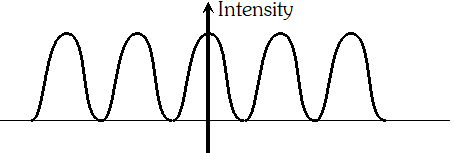(b) When one slit is closed, diffraction pattern is obtained on the screen.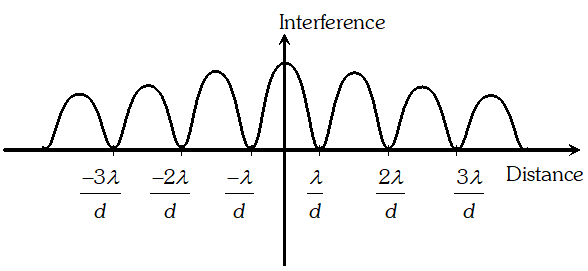Q. When viewing through a compound microscope, our eyes should be positioned not on the eye piece, but a short distance away from it for best viewing, why? How much should be that short distance between the eye and eye piece? [NCERT]
Ans. The image of objective lens in eye piece is called ‘eye ring’. All the rays from the object refracted by the objective go through the eye ring. Therefore, ideal position for our eyes for viewing is this eye ring only. When eye is too close to the eye piece, field of view reduces and eyes do not collect much of the light. The precise location of the eye ring would depend upon the separation between the objective and eye piece, and also on focal length of the eye piece.
Q. How does the resolving power of a compound microscope change on (i) decreasing the wavelength of light used, and (ii) Decreasing the diameter of its objective lens?
Ans. For a compound microscope resolving power $=\frac{2 \mu \sin \theta}{\lambda}$ (i) The resolving power increases on decreasing the wavelength of light. (ii) Resolving power does not change on decreasing the diameter of the objective lens.
Q. Draw a labelled ray diagram showing the formation of image of a distant object using an astronomical telescope in the normal adjustment position. Write down the expressions for it’s magnifying power and length.
Ans.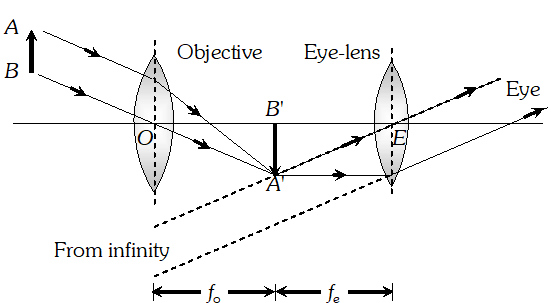In normal adjustment, final image if formed at $\infty$ Magnifying power $: m=\frac{f_{0}}{f_{e}},$ Length $L=f_{0}+f_{e}$
Q. Using the data given below, state as to which of the given lenses will you prefer to use as (i) an eyepiece, and (ii) an objective, to construct an astronomical telescope. Give reason for your answer.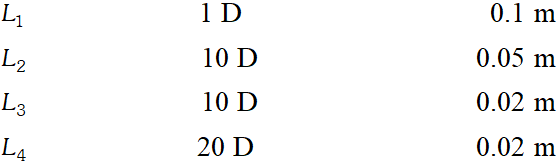Sol. For higher magnification of a telescope $f_{0}$ should be high and $f_{e}$ should be less. For higher resolving power of telescope aperture of objective should be high. Hence to construct an astronomical telescope (i) $L_{4}$ should be used as eyepiece because it's focal length and aperture is smallest (ii) $L_{1}$ should be used as objective because it's aperture is largest.
Q. Using the data given below, state as to which of the given lenses will you prefer to use as (i) an eye–piece, and (ii) an objective, to design a compound microscope. Give reason for your answer.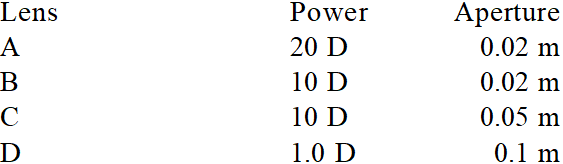Ans.  (i) Lens C should be used as eye piece because of it’s small focal length and comparatively larger aperture. (ii) Lens A should be used as objective because of it’s very small focal length and small aperture
Q. Does short sightedness (myopia) or long sightedness (hypermetropia) imply necessarily that the eye has partially lost its ability of accommodation ? If not, what might cause these defects of vision ? [NCERT]
Ans. No, a person may have normal ability of accommodation and yet he may be myopic or hypemetropic. Infact myopia arises when length of eye ball (from front to back) gets elongated and hypermetropia arises when length of eye ball gets shortened. However, when eye ball has normal length, but the eye lens loses partially its power of accommodation, the defect is called presbiopia.
Q.A person looking at a person wearing a shirt with a pattern comprising vertical and horizontal lines is able to see the vertical lines more distinctly than the horizontal ones. What is this defect due to ? How is such a defect of vision corrected? [NCERT]
Ans. This defect is called astigmatism. It arises because curvature of the cornea plus eye lens refracting system is not the same in different planes. As vertical lines are seen distinctly, the curvature in the vertical plane is enough, but in the horizontal plane, curvature is insufficient. This defect is removed by using a cylindrical lens with its axis along the vertical.
Q. Draw a labelled ray diagram for a refracting type astronomical telescope when final image formed at least distinct vision. How will its magnifying power be affected on increasing for its eye-piece (i) The focal length, and (ii) The aperture? Justify your answer
Ans.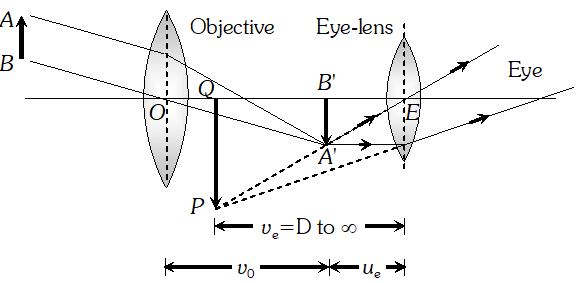Magnifying power ( $m$ ): magnifying power of telescope is $\frac{f_{0}}{f_{e}}\left(1+\frac{f_{e}}{D}\right)($ i) By increasing focal
Q. Draw a labelled ray diagram for a compound microscope. How will its resolving power be affected when (a) the frequency of light used to illuminate the object is increased, and (b) the focal length of the objective is increased. Justify your answer in each case.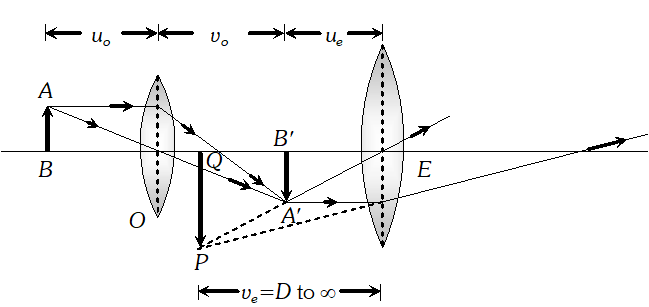Ans. Resolving power of a compound microscope $\frac{2 \mu \sin \theta}{\lambda}=\frac{2 \mu \sin \theta \times v}{c}$ $[c=v \lambda]$ (i) When the frequency of light $(v)$ increases, the resolving power also increases (ii) When $f_{0}$ is increased, resolving power does not change.
Q. Draw a labelled ray diagram to show the image formation in a reflecting type telescope. Write its two advantages over a refracting type telescope. On what factors does its resolving power depend?
Ans.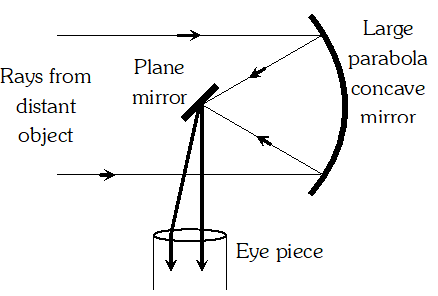Advantage : (i) There is no chromatic aberration as the objective is a mirror. (ii) Image is brighter compared to that in refracting type telescope. The resolving power of the telescope depends on the aperture of the objective and wavelength of light used.
Q. A myopic person has been using spectacles of power –1.0 dioptre distant vision. During old age, he also needs to use separate reading glasses of power +2.0 dioptre. Explain what may have happened [NCERT]
Ans. As the person is using spectacles of power –1.0 dioptre (i.e. focal length – 100 cm), the far point of the person is at 100 cm, Near the point of the eye might have been normal (i.e. 25 cm). The object at infinity produce virtual images at 100 cm (using spectacles). To see objects between 25 cm and 100 cm. the person uses the ability of accommodation of his eye lens. This ability is partially lost in old age. The near point of the eye may recede to 50 cm. He has, therefore to use glasses of suitable power for reading. Here, $u=-25 \mathrm{cm}, v=-50 \mathrm{cm}, f=?$ As $\frac{1}{v}-\frac{1}{u}=\frac{1}{f}: \quad \frac{-1}{50}+\frac{1}{25}=\frac{1}{f}$ $\frac{-1+2}{50}=\frac{1}{f}$ or $| f=50 \mathrm{cm}$ As $P=\frac{100}{f}=\frac{100}{50}=+2$ dioptre.
Q. A small telescope has an objective lens of focal length 140 cm and eye piece of focal length 5.0 cm. What is the magnifying power of telescope for viewing distant objects when (a) The telescope is in normal adjustment (i.e. when the final image is at infinity) (b) The final image is formed at the least distance of distinct vision (25 cm). [NCERT]
Ans. Here, $f_{0}=140 \mathrm{cm}, f_{e}=5.0 \mathrm{cm}$ Magnifying power $=?$ (a) In normal adjustment, Magnifying power $=\frac{f_{0}}{-f_{e}}=\frac{140}{-5}=-28.$ (b) When final image is at the least distance of distinct vision Magnifying power $=\frac{-f_{0}}{f_{e}}\left(1+\frac{f_{e}}{d}\right)=-\frac{140}{5}\left(1+\frac{5}{25}\right)=34.5$
Q. For a normal eye, the far point is at infinity and the near point of distinct vision is about 25 cm in front of the eye. The cornea of the eye provides a converging power of about 40 dioptre and the least converging power of eye lens behind the cornea is about 20 dioptre. From this rough data, estimate the range of accommodation of a normal eye. [NCERT]
Ans. To observe objects at infinity, the eye uses its least converging power = 40 + 20 = 60 D.  Distance between cornea/eye lens and retina = focal length of eye lens $=\frac{100}{P}=\frac{100}{60}=\frac{5}{3} \mathrm{cm}$ To focus an object at the near point $u=-25 \mathrm{cm} . v=5 / 3 \mathrm{cm} . f=?$ $\frac{1}{f}-\frac{1}{u}+\frac{1}{v}=\frac{1}{25}+\frac{3}{5}=\frac{1+15}{25}=\frac{16}{25} \Rightarrow f=\frac{25}{16} \mathrm{cm}$ Power $=\frac{100}{f}=\frac{100}{\frac{25}{16}}=64 D$ Power of eye lens $=64-40=24 D$ Hence range of accommodation of eye lens is roughly 20 to 24 dioptre.
Q. A beaker is filled with water to a height of 12.5 cm. The apparent depth of a needle lying at the bottom of the tank is measured by a microscope to be 9.4 cm. What is the refractive index of water ? If water is replaced by a liquid of refractive index 1.63 upto the same height, by what distance would the microscope have to be moved to focus on the needle again ? [NCERT]
Ans. $\begin{array}{ll}{\text { Sol. }} & {\text { Here, real depth }=12.5 \mathrm{cm}, \text { apparent depth }=9.4} \\ {} & {c m ; \mu=?}\end{array}$ As $\mu=\frac{\text { real depth }}{\text { apparent depth }} \Rightarrow \mu=\frac{12.5}{9.4}=1.33$ Now, in the second case, $\mu=1.63,$ real depth $=$ $12.5 \mathrm{cm},$ apparent depth $h^{\prime}=?$ $\therefore 1.63=\frac{12.5}{h^{\prime}}, h^{\prime}=\frac{12.5}{1.63}=7.67 \mathrm{cm}$ $\therefore$ Distance through which microscope has to be moved up $=9.4-7.67=1.73 \mathrm{cm}$
Q. A compound microscope consists of an objective lens of focal length 2.0 cm and an eye piece of focal length 6.25 cm, separated by a distance of 15 cm. How far from the objective should an object be placed in order to obtain the final image at (a) The least distance of distinct vision (25 cm) (b) Infinity? What is the magnifying power of the microscope in each case? [NCERT]
Ans. Here, $f_{0}=2.0 \mathrm{cm}, f_{e}=6.25 \mathrm{cm}, u_{0}=?$ (a) $v_{e}=-25 \mathrm{cm}$ As $\frac{1}{v_{e}}-\frac{1}{u_{e}}=\frac{1}{f_{e}}$ $\therefore \frac{1}{u_{e}}=\frac{1}{v_{e}}-\frac{1}{f_{e}}=\frac{1}{-25}-\frac{1}{6.25}=\frac{-5}{25} \Rightarrow u_{e}=-5 \mathrm{cm}$ As distance between objective and eye piece = 15 cm. $\therefore v_{0}=15-5=10 \mathrm{cm}$ As $\frac{1}{v_{0}}-\frac{1}{u_{0}}=\frac{1}{f_{0}}$ $\frac{1}{u_{0}}=\frac{1}{v_{0}}-\frac{1}{f_{0}}=\frac{1}{10}-\frac{1}{2}=\frac{1-5}{10}$ $u_{0}=\frac{-10}{4}=-2.5 \mathrm{cm}$ Magnifying power$=\frac{v_{0}}{\left|u_{0}\right|}\left(1+\frac{d}{f_{e}}\right)=\frac{10}{2.5}\left(1+\frac{25}{6.25}\right)=20$ (b) As $v_{e}=\infty,-u_{e}=f_{e}=6.25 \mathrm{cm}$ $\therefore v_{0}=15-6.25=8.75 \mathrm{cm}$ As $\frac{1}{v_{0}}-\frac{1}{u_{0}}=\frac{1}{f_{0}}$ $\frac{1}{u_{0}}=\frac{1}{v_{0}}-\frac{1}{f_{0}}=\frac{1}{8.75}-\frac{1}{2.0}=\frac{2-8.75}{17.5}$ $u_{0}=\frac{-17.5}{6.75}=-2.59$ magnifying power $=\frac{v_{0}}{\left|u_{0}\right|} \times\left(1+\frac{d}{f_{e}}\right)=\frac{v_{0}}{\left|u_{0}\right|} \times \frac{d}{\left|u_{e}\right|}$ $=\frac{8.75}{2.59} \times \frac{25}{6.25}=13.51$
Q. A person with a normal near point 25 cm using a compound microscope with an objective of focal length 8.0 mm and an eye piece of focal length 2.5 cm can bring an object placed 9.0 mm from the objective in sharp focus. What is the separation between the two lenses ? How much is the magnifying power of the micoscope [NCERT]
Ans. Here $D=25 \mathrm{cm}, f_{0}=8.0 \mathrm{mm}=0.8 \mathrm{cm}, f_{e}=2.5 \mathrm{cm},$ $u=-9.0 \mathrm{mm}=-0.9 \mathrm{cm}$ As $\frac{1}{v_{e}}-\frac{1}{u_{e}}=\frac{1}{f_{e}} \Rightarrow \frac{1}{u_{e}}=\frac{1}{v_{e}}-\frac{1}{f_{e}}\left(v_{e}=-D=-25 \mathrm{cm}\right)$ $\frac{1}{u_{e}}=\frac{1}{-25}-\frac{1}{2.5}=\frac{-1-10}{25}=-\frac{11}{25}=-\frac{25}{11}=-2.27 \mathrm{cm}$ Again $\frac{1}{v_{0}}-\frac{1}{u_{0}}=\frac{1}{f_{0}}$ $\frac{1}{v_{0}}=\frac{1}{f_{0}}+\frac{1}{u_{0}}=\frac{1}{0.8}+\frac{1}{-0.9}=\frac{0.9-0.8}{0.72}=\frac{0.1}{0.72}$ $v_{0}=\frac{0.72}{0.1}=7.2 \mathrm{cm}$ $\therefore$ Separation between the two lenses $=\left|u_{e}\right|+v_{0}=2.27+7.2=9.47 \mathrm{cm}$ Magnifying power$=\frac{v_{0}}{\left|u_{0}\right|}\left(1+\frac{d}{f_{e}}\right)=\frac{7.2}{0.9}\left(1+\frac{25}{2.5}\right)=88$
Q. (a) A giant refracting telescope at an observatory has an objective lens of focal length 15 m. If an eye piece of focal length 1.0 cm is used, what is the angular magnification of telescope ? (b) If this telescope is used to view the moon, what is the diameter of the image of the moon formed by the objective lens ? The diamete r of the moon is $3.48 \times 10^{6}$ m and radius of lunar orbit is is $3.8 \times 10^{8}$ [NCERT]
Ans. Here, $f_{0}=15 m, f_{e}=1.0 \mathrm{cm}=10^{-2} \mathrm{m}$ Angular magnification $=\frac{f_{0}}{f_{e}}=\frac{15}{10^{-2}}=1500$ (b) If $d$ is diameter of the image, then angle subtended by diameter of moon = $\frac{3.48 \times 10^{6}}{3.8 \times 10^{8}}$ and, angle subtended by image $=\frac{d}{f_{0}}=\frac{d}{15}$ $\therefore \frac{d}{15}=\frac{3.48 \times 10^{6}}{3.8 \times 10^{8}}$ $d=\frac{3.48 \times 15 \times 10^{-2}}{3.8}=13.73 \times 10^{-2} \mathrm{m}=13.73 \mathrm{cm}$
Q. A compound microscope with an objective of 1.0 cm focal length and an eye-piece of 2.0 cm focal length has a tube length of 20 cm. Calculate the magnifying power of the microscope, if the final image is formed at the near point of the eye. [CBSE 2004]
Ans. $\quad m=-\frac{v_{0}}{u_{0}}\left(1+\frac{D}{f_{e}}\right)$ and $L=v_{0}+u_{e}$ It is given $f_{0}=1 \mathrm{cm}, f_{e}=2 \mathrm{cm}, L=20 \mathrm{cm}$ By using lens formula for eye lens.$\frac{1}{f_{e}}=\frac{1}{v_{e}}-\frac{1}{u_{e}} \Rightarrow \frac{1}{+2}=\frac{1}{-25}-\frac{1}{u_{e}} \Rightarrow u_{0}=\frac{-50}{27} \mathrm{cm}$ $v_{0}=\frac{490}{27} \mathrm{cm}$ Again by using lens formula for objective lens $\frac{1}{f_{0}}=\frac{1}{v_{0}}-\frac{1}{u_{0}} \Rightarrow \frac{1}{+1}=\frac{1}{490 / 27}-\frac{1}{u_{0}} \Rightarrow u_{0}=-\frac{490}{463} \mathrm{cm}$ Hence $m=-\frac{490 / 27}{490 / 463}\left(1+\frac{25}{2}\right) \Rightarrow m=-231.5$ Method $\mathbf{I I}: m=-\frac{v_{0}}{u_{0}}\left(1+\frac{D}{f_{e}}\right)$ As the object lies very close to $f_{0}$, therefore $u_{0} \approx f_{0}$ As image formed by objective is very close to eye lens therefore $v_{0} \approx L=$ length of microscope tube Hence $m \approx-\frac{L}{f_{0}}\left(1+\frac{D}{f_{e}}\right)$ on putting value $m \approx-\frac{20}{1}\left(1+\frac{25}{2}\right) \approx-270$
Q. A man with normal near point (25 cm) reads a book with small print using a magnifying glass: a thin convex lens of focal length 5 cm. (a) What are the closest and farthest distances at which he can read the book, when viewing through the magnifying glass? (b) What is the maximum and the minimum angular magnifications (magnifying powers) possible using the above simple microscope? [NCERT]
Ans. (a) Here, $f=5 \mathrm{cm}, u=?$ For the closest distance; $v=-25 \mathrm{cm}$ As $\frac{1}{f}=\frac{1}{v}-\frac{1}{u} \Rightarrow \frac{1}{u}=\frac{1}{v}-\frac{1}{f}=\frac{1}{-25}-\frac{1}{5}=\frac{-1-5}{25}$ $\Rightarrow u=\frac{25}{-6}=-4.2$ This is the closest distance at which he can read the book. For the farthest distance, $v^{\prime}=\infty, u^{\prime}=?$ As $\frac{1}{f}=\frac{1}{v^{\prime}}-\frac{1}{u^{\prime}} \Rightarrow \frac{1}{u^{\prime}}=\frac{1}{v^{\prime}}-\frac{1}{f}=\frac{1}{\infty}-\frac{1}{5}=-\frac{1}{5} \Rightarrow$ $u^{\prime}=-5 c m$ This the farthest distance at which he can read the book (b) Maximum angular magnification $m=\frac{d}{u}=\frac{25}{25 / 6}=6$ Minimum angular magnification $m=\frac{d}{u^{i}}=\frac{25}{5}=5$
Click Here for Detailed Notes of any chapter. `
eSaral provides you complete edge to prepare for Board and Competitive Exams like JEE, NEET, BITSAT, etc. We have transformed classroom in such a way that a student can study anytime anywhere. With the help of AI we have made the learning Personalized, adaptive and accessible for each and every one. Visit eSaral Website to download or view free study material for JEE & NEET. Also get to know about the strategies to Crack Exam in limited time period.